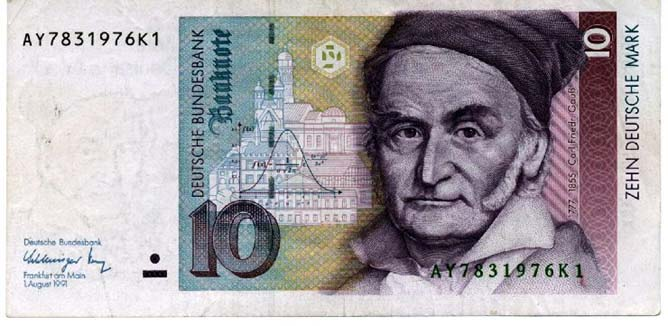# Lecture Notes on Differential Geometry## Volume I: Curves and Surfaces

• Lecture Notes 0

Basics of Euclidean Geometry, Cauchy-Schwarz inequality.

• Lecture Notes 1

Definition of curves, examples, reparametrizations, length, Cauchy's integral formula, curves of constant width.

• Lecture Notes 2

Isometries of Euclidean space, formulas for curvature of smooth regular curves.

• Lecture Notes 3

General definition of curvature using polygonal approximations (Fox-Milnor's theorem).

• Lecture Notes 4

Curves of constant curvature, the principal normal, signed curvature, turning angle, Hopf's theorem on winding number, fundamental theorem for planar curves.

• Lecture Notes 5

Osculating circle, Kneser's Nesting Theorem, total curvature, convex curves.

• Lecture Notes 6

The four vertex theorem, Shur's arm lemma, isoperimetric inequality.

• Lecture Notes 7

Torsion, Frenet-Seret frame, helices, spherical curves.

• Lecture Notes 8

Definition of surface, differential map.

• Lecture Notes 9

Gaussian curvature, Gauss map, shape operator, coefficients of the first and second fundamental forms, curvature of graphs.

• Lecture Notes 10

Interpretations of Gaussian curvature as a measure of local convexity, ratio of areas, and products of principal curvatures.

• Lecture Notes 11

Intrinsic metric and isometries of surfaces, Gauss's Theorema Egregium, Brioschi's formula for Gaussian curvature.

• Lecture Notes 12

Gauss's formulas, Christoffel symbols, Gauss and Codazzi-Mainardi equations, Riemann curvature tensor, and a second proof of Gauss's Theorema Egregium.

• Lecture Notes 13

The covariant derivative and Lie bracket; Riemann curvature tensor and Gauss's formulas revisited in index free notation.

• Lecture Notes 14

The induced Lie bracket on surfaces. Self adjointness of the shape operator, Riemann curvature tensor of surfaces, Gauss and Codazzi Mainardi equations, and Theorema Egregium revisited.

• Lecture Notes 15

Definition of geodesic curvature, and the proof that it is intrinsic.

• Lecture Notes 16

The Gauss-Bonnet theorem, and its applications.

## Volume II: Manifolds

• Lecture Notes 1

Review of basics of Euclidean Geometry and Topology. Proofs of the Cauchy-Schwartz inequality, Heine-Borel and Invariance of Domain Theorems.

• Lecture Notes 2

Definition of manifolds and some examples.

• Lecture Notes 3

Immersions and Embeddings. Proof of the embeddibility of comapct manifolds in Euclidean space.

• Lecture Notes 4

Definition of differential structures and smooth mappings between manifolds.

• Lecture Notes 5

Definition of Tangent space. Characterization of tangent space as derivations of the germs of functions. Differential map and diffeomorphisms.

• Lecture Notes 6

Proofs of the inverse function theorem and the rank theorem.

• Lecture Notes 7

Smooth submanifolds, and immersions. Proof of the smooth embeddibility of smooth manifolds in Euclidean space. Tangent Bundles.

• Lecture Notes 8

Proof of Whitney's 2n+1 embedding theorem.

• Lecture Notes 9

Regular values, proof of fundamental theorem of algebra, Smooth manifolds with boundary, Sard's theorem, and proof of Brouwer's fixed point theorem.

• Lecture Notes 10

Proof of Sard's theorem (not yet typeset, but contains some exercises).

• Lecture Notes 11

Various definitions of orientability and the proof of their equivalence. Proof of the nonorientability of the Mobius strip and the nonembeddability of the real projective plane in R3. Proof that RPn is oreintable for n odd and is not orientable for n even.

• Lecture Notes 12

Definition of a Riemannian metric, and examples of Riemannian manifolds, including quotients of isometry groups and the hyperbolic space. The notion of distance on a Riemannian manifold and proof of the equivalence of the metric topology of a Riemannian manifold with its original topology.

• Lecture Notes 13

Integration on manifolds, definition of volume, and proof of the existence of partition of unity.

• Lecture Notes 14

Connections, covariant derivative, parallel translations, Foucault's pendulum, and geodesics. Proof of the existence and uniqueness of geodesics.

• Lecture Notes 15

Riemannian connections, brackets, proof of the fundamental theorem of Riemannian geometry, induced connection on Riemannian submanifolds, reparameterizations and speed of geodesics, geodesics of the Poincare's upper half plane.

• Lecture Notes 16

Exponential map and geodesic flow.